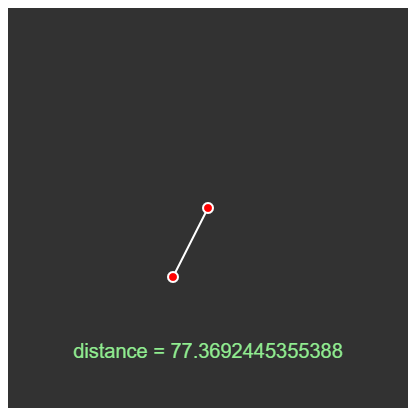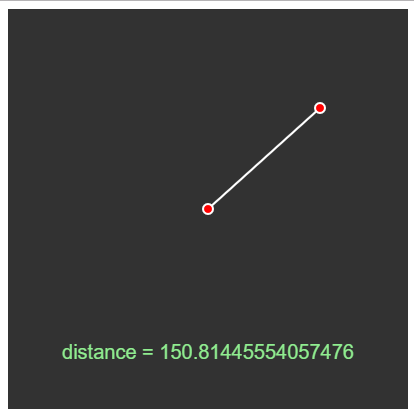GFG App
Open AppBrowser
Continue

# p5.js | dist() Function

The dist() function calculates the Euclidean distance in 2D or 3D. Means the p5.js | dist() Function is used to measure the distance between two points in 2D or 3D. Below is the formula for distance in 2D and 3D.

• 2D formula:• 3D formula:Syntax:

dist(x1, y1, x2, y2)

dist(x1, y1, z1, x2, y2, z2)


Parameters:

• x1: These parameters holds the x-coordinate of the first point.
• y1: These parameters hold the y-coordinate of the first point.
• z1: These parameters hold the z-coordinate of the first point.
• x2: These parameters hold the x-coordinate of the second point.
• y2: These parameters hold the y-coordinate of the second point.
• z2: These parameters hold the z-coordinate of the second point.

Return value: Distance between two points.
Example: This example calculates and prints the distance between a fixed point and the cursor.

 function setup() {      // Create a canvas      createCanvas(400, 400);  }     function draw() {      // set background color      background(50);      // coordinates of the fixed point      var x1 = 200;      var y1 = 200;      // coordinates of the cursor      var x2 = mouseX;      var y2 = mouseY;      // set line color and weight      stroke(255);      strokeWeight(2);      // draw a line connecting 2 points      line(x1, y1, x2, y2);      fill("red");      // draw a circle centered at each point      ellipse(x1, y1, 10);      ellipse(x2, y2, 10);      // calculate the distance between 2 points      d = dist(x1, y1, x2, y2);      noStroke();      fill("lightgreen");      // set text size and alignment      textSize(20);      textAlign(CENTER);      // display the distance calculated      text("distance = "+ str(d), 200, 350);  }

Output:Online editor: https://editor.p5js.org/
Environment Setup: https://www.geeksforgeeks.org/p5-js-soundfile-object-installation-and-methods/

Reference: https://p5js.org/reference/#/p5/dist

My Personal Notes arrow_drop_up